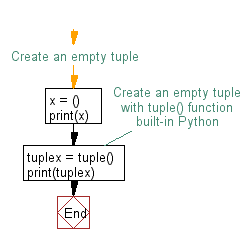﻿ Python: Create a tuple - w3resource# Python Exercise: Create a tuple

## Python tuple: Exercise-1 with Solution

Write a Python program to create a tuple.

Sample Solution:-

Python Code:

``````#Create an empty tuple
x = ()
print(x)
#Create an empty tuple with tuple() function built-in Python
tuplex = tuple()
print(tuplex)
```
```

Sample Output:

```()
()
```

Flowchart:## Visualize Python code execution:

The following tool visualize what the computer is doing step-by-step as it executes the said program:

Python Code Editor:

Have another way to solve this solution? Contribute your code (and comments) through Disqus.

What is the difficulty level of this exercise?

Test your Programming skills with w3resource's quiz.

﻿

## Python: Tips of the Day

Decapitalizes the first letter of a string:

Example:

```def tips_decapitalize(s, upper_rest=False):
return s[:1].lower() + (s[1:].upper() if upper_rest else s[1:])
print(tips_decapitalize('PythonTips'))
print(tips_decapitalize('PythonTips', True))
```

Output:

```pythonTips
pYTHONTIPS
```